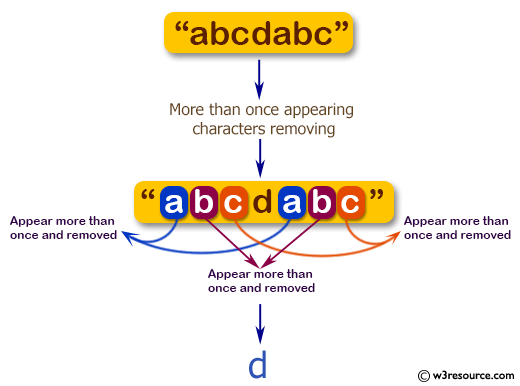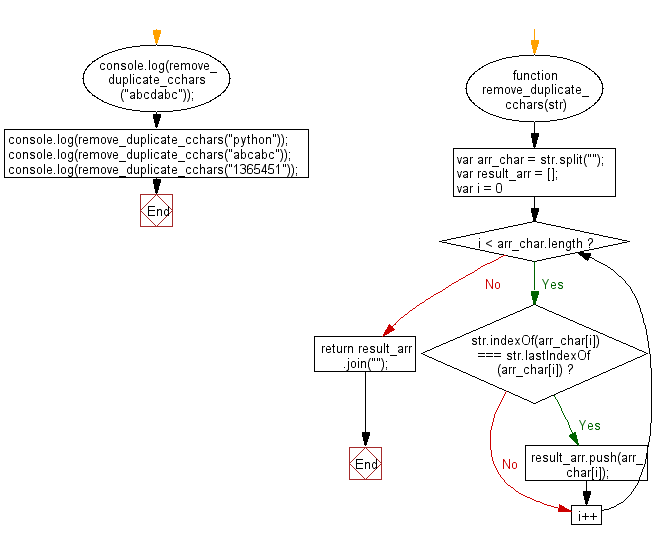# JavaScript: Remove all characters from a given string that appear more than once

## JavaScript Basic: Exercise-135 with Solution

Write a JavaScript program to remove all characters from a given string that appear more than once.

Pictorial Presentation:Sample Solution:

HTML Code:

``````<!DOCTYPE html>
<html>
<meta charset="utf-8">
<meta name="viewport" content="width=device-width">
<title>Remove all characters from a given string that appear more than once</title>
<body>

</body>
</html>
```
```

JavaScript Code:

``````function remove_duplicate_cchars(str) {
var arr_char = str.split("");
var result_arr = [];

for (var i = 0; i < arr_char.length; i++) {
if (str.indexOf(arr_char[i]) === str.lastIndexOf(arr_char[i]))
result_arr.push(arr_char[i]);
}

return result_arr.join("");
}
console.log(remove_duplicate_cchars("abcdabc"));
console.log(remove_duplicate_cchars("python"));
console.log(remove_duplicate_cchars("abcabc"));
console.log(remove_duplicate_cchars("1365451"));
``````

Sample Output:

```d
python

364
```

Flowchart:ES6 Version:

``````function remove_duplicate_cchars(str) {
const arr_char = str.split("");
const result_arr = [];

for (let i = 0; i < arr_char.length; i++) {
if (str.indexOf(arr_char[i]) === str.lastIndexOf(arr_char[i]))
result_arr.push(arr_char[i]);
}

return result_arr.join("");
}
console.log(remove_duplicate_cchars("abcdabc"));
console.log(remove_duplicate_cchars("python"));
console.log(remove_duplicate_cchars("abcabc"));
console.log(remove_duplicate_cchars("1365451"));
``````

Live Demo:

See the Pen javascript-basic-exercise-135 by w3resource (@w3resource) on CodePen.

Improve this sample solution and post your code through Disqus

What is the difficulty level of this exercise?

Test your Programming skills with w3resource's quiz.

﻿

## JavaScript: Tips of the Day

Returns the sum of the powers of all the numbers from start to end (both inclusive)

Example:

```const sumPower = (end, power = 2, start = 1) =>
Array(end + 1 - start)
.fill(0)
.map((x, i) => (i + start) ** power)
.reduce((a, b) => a + b, 0);
console.log(sumPower(10)); // 385
console.log(sumPower(10, 3)); // 3025
console.log(sumPower(10, 3, 5)); // 2925
```

Output:

```385
3025
2925
```